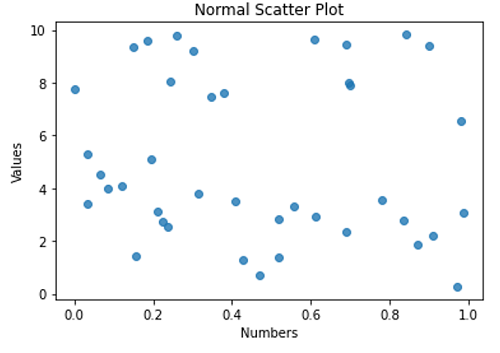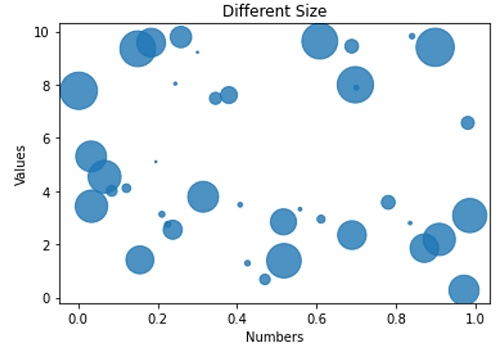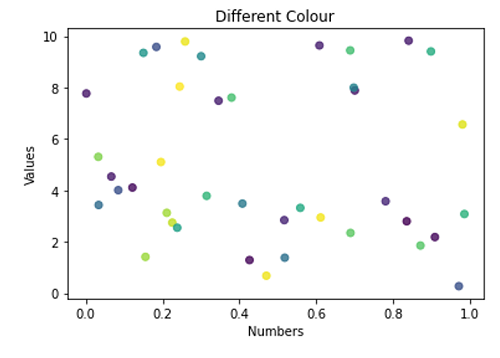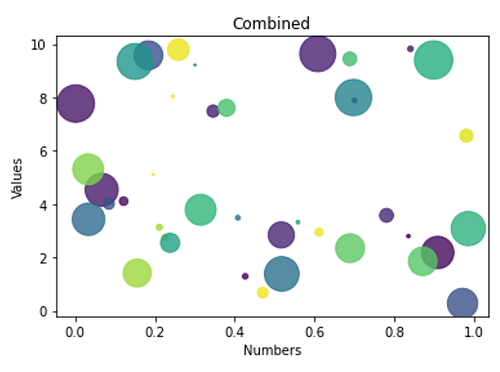# Python | Scatter Plot

Python | Scatter Plot: In this tutorial, we are going to learn about the scatter plot and its implementation with examples.
Submitted by Anuj Singh, on July 07, 2020

Inherited from the Dot Plots, Scatter plots are of very similar types. It provides a power of different features for every individual point. We are allowed to vary the size, color, and other properties of each data point and which makes data more friendly to visualize.

## 1) Normal Scatter Plot

Syntax:

```plt.scatter(x, y, alpha=0.8)
```

Parameter(s):

• x, y - data points in an array
• Alpha = 0.8 - opaque value (0 for transparent and 1 for opaque)## 2) Scatter Plot with variable size

Syntax:

```plt.scatter(x, y, s=area, alpha=0.8)
```

Parameter(s):

• x, y - data points in an array
• Alpha = 0.8 - opaque value (0 for transparent and 1 for opaque)
• s = area - the size of each point in terms of area## 3) Dash Point Plots with color code Green, Red, Blue Yellow

Syntax:

```plt.scatter(x, y, c=colors, alpha=0.8)
```

Parameter(s):

• x, y - data points in an array
• Alpha = 0.8 - opaque value (0 for transparent and 1 for opaque)
• c = colors - colors of each point## 4) Star Point Plot with Color Code Blue, Green, Red, Yellow

Syntax:

```plt.scatter(x, y, c=colors, s=area, alpha=0.8)
```

Parameter(s):

• x, y - data points in an array
• Alpha = 0.8 - opaque value (0 for transparent and 1 for opaque)
• c = colors - colors of each point
• s = area - size of each point in terms of areaApplications:

1. Machine Learning
2. Monte Carlo Simulation
3. Case Studies
4. Probabilistic Models

## Python code to demonstrate example of scatter plot

```import numpy as np
import matplotlib.pyplot as plt

N = 40
x = np.random.rand(N)
y = np.random.rand(N)*10

# random colour for points, vector of length N
colors = np.random.rand(N)

# area of the circle, vectoe of length N
area = (30 * np.random.rand(N))**2
# 0 to 15 point radii

# a normal scatter plot with default features
plt.scatter(x, y, alpha=0.8)
plt.xlabel('Numbers')
plt.ylabel('Values')
plt.title('Normal Scatter Plot')
plt.show()

# a scater plot with different size
plt.figure()
plt.scatter(x, y, s=area, alpha=0.8)
plt.xlabel('Numbers')
plt.ylabel('Values')
plt.title('Different Size')
plt.show()

# a scatter plot with different collour
plt.figure()
plt.scatter(x, y, c=colors, alpha=0.8)
plt.xlabel('Numbers')
plt.ylabel('Values')
plt.title('Different Colour')
plt.show()

# A combined Scatter Plot
plt.figure()
plt.scatter(x, y, s=area, c=colors, alpha=0.8)
plt.xlabel('Numbers')
plt.ylabel('Values')
plt.title('Combined')
plt.show()
```

Output:

```Output is as figure
```